## Spectral Decomposition

Diagonalization of a real symmetric matrix is also called spectral decomposition, or Schur Decomposition. Given a square symmetric matrix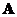, the matrix can be factorized into two matrices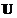and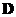. Matrixis an orthogonal matrix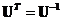. Matrixis a diagonal matrix . Spectral decomposition is matrix factorization because we can multiply the matrices to get back the original matrix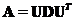.

The interactive program below yield three matrices,and matrix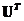and also gives you feedback on. Random example will generate random symmetric matrix.

Yes, this program is a free educational program!! Please don't forget to tell your friends and teacher about this awesome program!